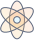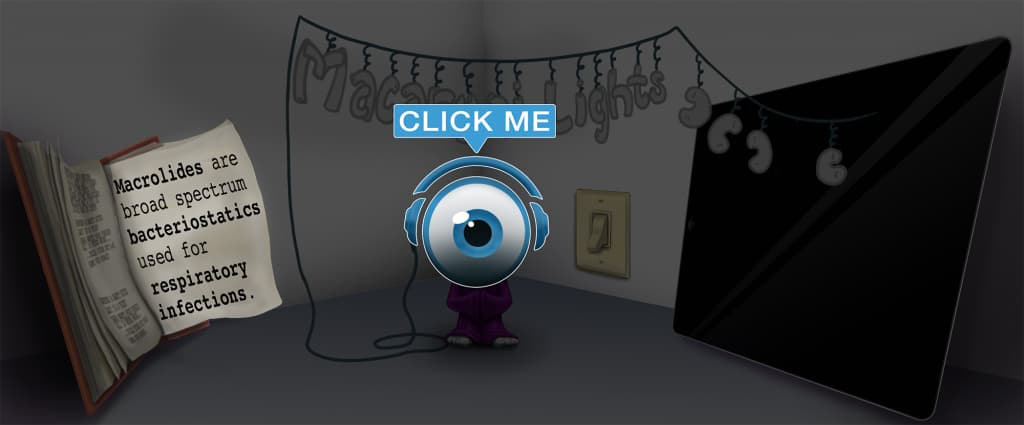# Master Infusions Prescribed by Unit Dosage per Hour with Picmonic for Nursing RN

## With Picmonic, facts become pictures. We've taken what the science shows - image mnemonics work - but we've boosted the effectiveness by building and associating memorable characters, interesting audio stories, and built-in quizzing.

### Infusions Prescribed by Unit Dosage per Hour

In-fuse IV with Hour-glassPicmonic
When an infusion is prescribed by unit dosage per hour, you will need to determine the amount of milliliters (mL) to infuse over each hour. To determine the unit dosage per hour, there is a two-step process used to calculate these problems. In step one, you will need to determine the amount of medication (units) per milliliter. This is done by calculating the known amount of medication in solution divided by total volume of diluent, which equals the amount of medication per milliliter. In step two, you will calculate the infusion rate (milliliters per hour). To do this, you will take the dose ordered, divided by the concentration per milliliter (your answer from step one), which equals the infusion rate, or mL/hour.
4 KEY FACTS
STEP 1
Determine Amount of Medication per 1mL
D-terminator with Med-bottle and (1) Wand Millionaire-liter-of-cola

The first step of the equation is to calculate the amount of medication per 1 mL. This will make it easier to calculate the mL per hour in the next step.

Known Amount of Medication in Solution/Total Volume of Diluent = Amount of Medication per Milliliter
Med-bottle in Solution / Volume-cup of Dissolving-liquid = Med-bottle and Millionaire-liter-of-cola

The first calculation is to divide the known amount of units in the solution by the total volume of diluent. Example: The available IV bag of medication is 25,000 units of heparin in 500 mL D5W; the equation would be 25,000 units/500mL= 50 units/1mL.

STEP 2
Calculate mL per Hour
Calculator Millionaire-liter-of-cola and Hour-glass

In the second step, you calculate how many milliliters to give over an hour. This is done by using the amount of units per mL which was calculated in step 1.

Dose per Hour Desired/Concentration per Milliliter = Infusion Rate (mL/hour)
Dose and Hour-glass / Concentration Millionaire-liter-of-cola = In-fuse with Millionaire-liter-of-cola and Hour-glass

In this equation you take the dose per hour desired; which is the prescribed amount, divided by the concentration per milliliter; which is the amount of units/hour that was calculated in step 1 (50 units/1mL). This will give you the infusion rate or mL/hour.

Unlock all 4 facts & definitions with Picmonic Free!

## Take the Infusions Prescribed by Unit Dosage per Hour Quiz

Picmonic's rapid review multiple-choice quiz allows you to assess your knowledge.

## Picmonic for Nursing RN CoversRegistered Nurse (RN)
1,100+ Videos
13,000+ Facts

## Our Story Mnemonics Increase Mastery and Retention

Memorize facts with phonetic mnemonics

Unforgettable characters with concise but impactful videos (2-4 min each)

### Ace YourRegistered Nurse (RN)Classes & Exams with Picmonic:

#### Over 1,210,000 students use Picmonic’s picture mnemonics to improve knowledge, retention, and exam performance.